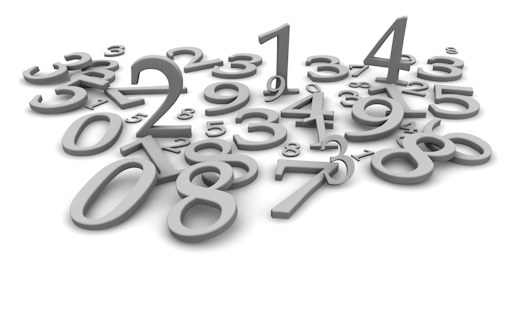Web pages: (Maintained by) Oliver Couto

Email:samson@celebrating-mathematics.com

Last Updated:

PARAMETRIC EQUATIONS FOR DEGREE TWO, THREE, FOUR, FIVE, SIX & SEVEN

Readers are welcome to send in their comments & suggestions to the above mentioned email contact info. Also they can contribute their mathematical articles & news about related mathematical developments.

Part 1. Misc. Identities Degree 2,3,4,5,6 & 7 (To be posted)

Part 2. Sums of Squares

Part 3. Third Powers

Part 4. Fourth Powers

Part 5. Fifth Powers

Part 6. Sixth Powers

Part 7. Seventh Powers

Part 1. Well known Miscellanous Identities:  (Degrees  2,3,4,&5)

Second degree

a = n2 -m2, b=2mn, c=m2+n2

Third degree

3m2+5mn-5n2, 4m2-4mn+6n2, 5m2-5mn-3n2; 6m2-4mn+4m2

Fourth degree

4-1-5 parametric solution

(2x2+12xy-6y2)4 + (2x2-12xy-6y2)4 + (4x2-12y2)4 + (4x2+12y2)4 + (3x2+9y2)4 = 54(x2+3y2)4

Fifth degree

((75y5-x5), (x5+25y5), (x5-25y5), (10x3y2), (50xy4))^5= (x5+75y5)^5

Catogeries of Equations (For future Postings)

 Sum of two squares 1. x2+y2 = zk 2. x2+y2 = z2+1 3. x2+y2 +1= z2 4. x2+y2 = z2+nt2 5. x2+y2 = z2+tk 6. x2+y2 = mz2+nt2 7. c1(x2+ny2) = c2(z2+nt2) 9. mx2+ny2 = mz2+nt2

 Sums of three squares 1. x2+y2+z2 = tk 2. x2+y2+z2 = u2+v2 3. (x2-1)(y2-1) = (z2-1)2 4. x2+y2+z2 = u2+v2+w2 5. x2+y2+z2 = (u2+v2+w2) 6. x2+y2+z2 = 3xyz

 Sums of four or five squares 1. a2+b2+c2+d2 = ek 2. a2+b2+c2+d2 = e2+f2 3. a2+b2+c2+d2 = e2+f2+g2 4. a2+b2+c2+d2 = e2+f2+g2+h2 5. a2+b2+c2+d2+e2 = f2

Sums of cubes

1. x3+y3 = z3

2. x3+y3+z3+t3 = 0

5. x3+y3+z3 = (m)3

6. x3+y3+z3 = (w)3

7. x3+y3 = 2(z3+t3)

8. w3+x3+y3+z3 = nt3

9. x3+y3+z3 = t2

10. xk+yk+zk = {p2, q3}, k =2,3

11. xk+yk+zk = tk+uk+vk,  k = 1,3

12. xk+yk+zk = tk+uk+vk,  k = 2,3

---------------------------------------

Sum of quartics:

1. a4+b4 = c4+d4

2. x4+y4 = z4+nt4

3. u4+nv4 = x4+y4+nz4

4. u4+v4 = x4+y4+nz4

5. x4+y4+z4 = ntk

6. ak+bk+ck = dk+ek+fk,  k = 2,4

 7 . ak+bk+ck = 2dk+ek,  k = 2,4 8. ak+bk+ck = dk+ek+fk,  k = 2,3,4 9. v4+x4+y4+z4 = ntk 10. v4+x4+y4+z4 = w4 11. vk+xk+yk+zk = ak+bk+ck+dk,  k = 2,4 12. 2(v4+x4+y4+z4) = (v2+x2+y2+z2)2 13. x1k+x2k+x3k+x4k+x5k = y1k+y2k+y3k, k = 1,2,3,4 14. x1k+x2k+x3k+x4k+x5k = y1k+y2k+y3k+y4k+y5k, k = 1,2,3,4 15. x14+x24+xm4=w^4   (m greater than 4)

## Index of updates:

Articles by Oliver Couto:

10) Sum of four ( n th ) powers"

11) Equal sums of four nth powers

12) Sums of fourth powers & second powers equal to zero

i) Quintic Equation